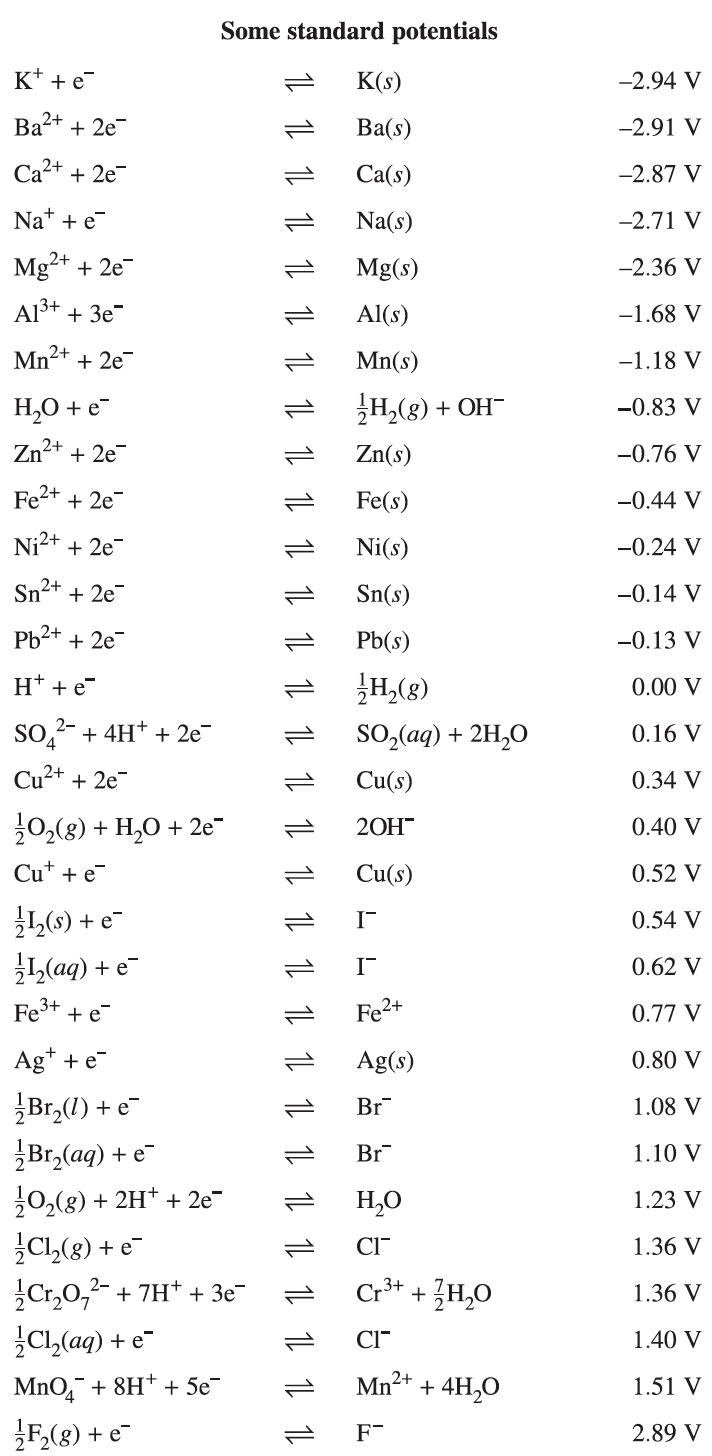# Redox Reactions

This is part of Year 11 HSC Chemistry course under the topic of Predicting Reactions of Metals

### HSC Chemistry Syllabus

• Apply the definitions of oxidation and reduction in terms of electron transfer and oxidation numbers to a range of reduction and oxidation (redox) reactions
• Construct relevant half-equations and balanced overall equations to represent a range of redox reactions
• Predict the reaction of metals in solutions using the table of standard reduction potentials
• Predict the spontaneity of redox reactions using the value of cell potentials

### Redox Reactions

This video explores what reaction reactions are? It explains the acronym OILRIG which refers to oxidation being the loss of electrons, while reduction is the gain of electrons.

### What is Redox?

Redox chemistry is all about the movement of electrons between different species in a reaction. To break it down:

• Reduction refers to the gain of electrons. For example, the transformation of iron ions into solid iron showcases reduction as iron accepts electrons to neutralise its charge.
• Oxidation means the loss of electrons. Consider magnesium turning into magnesium ions and releasing electrons; this process exemplifies oxidation.

These two processes always occur together – where one element loses electrons (oxidation) another gains them (reduction).

An easy mnemonic to help you remember the differences between oxidation and reduction is "OILRIG" which stands for "oxidation is loss, reduction is gain". This will help you identify whether a reaction involves oxidation or reduction, such as when magnesium loses electrons, it's undergoing oxidation.

### Half-Equations in Redox

Redox reactions can be split into half-equations, which separately describe the oxidation and reduction occurring in a reaction. Consider the following reaction between zinc and dilute acid:

$$Zn(s) + 2H^+ \rightarrow Zn^{2+}(aq) + H_2(g)$$

In this reaction, zinc has lost 2 electrons to form the Zn^{2+} ion while each hydrogen has gained one electron to form H_2. This transfer of electrons can be demonstrated by the following equations:

$$Zn \rightarrow Zn^{2+} + 2e^–$$

$$2H^+ + 2e^– \rightarrow H_2$$

The top half of the equation is the oxidation half equation since we know that the zinc has lost electrons to ionise, and the bottom half is the reduction half equation since hydrogen has gained electrons to become hydrogen gas.

When the we add the two equations together, we can see that there are 2 electrons on both sides of the equation

$$Zn + 2H^+ + 2e^– \rightarrow Zn^{2+} + 2e^– + H_2$$

We can then cross out the two electrons on both sides of the equation to provide us with the original net equation.

$$Zn(s) + 2H^+ \rightarrow Zn^{2+}(aq) + H_2(g)$$

It is important to know that in any redox reaction, there is an oxidising agent (which undergoes reduction) and a reducing agent (which undergoes oxidation). In the case of our two half equations, because zinc is undergoing oxidation, it must be the reducing agent which is causing the hydrogen ions to undergo reduction. Thus the reducing agent is also called the 'reductant'. This is true for the opposite scenario as hydrogen in the oxidising agent, also called the 'oxidant'

### Reduction Potentials

The reduction potential table is a key tool. It ranks substances based on their ability to gain electrons, which is indicated by their electrical potential. Substances with a higher reduction potential are more likely to undergo reduction, a principle crucial for predicting the outcome of chemical reactions.

### Understanding Reactivity

The reactivity of metals is linked to their position in the reduction potential table. More reactive metals, which are easily oxidised are found at the top of the table, like potassium (K), while more easily reduced substances are found further down, like silver (Ag).### Spontaneity

The concept of spontaneity in reaction tells us whether a reaction will proceed with or without external energy. Reduction potentials are used help us predict this. Now let's consider the reaction between copper and silver ions.

$$Cu(s) + Ag^+(aq) \rightarrow Cu^{2+}(aq) + Ag(s)$$

The half equations of this equation are:

$$Cu(s) \rightarrow Cu^{2+} + 2e^–$$

$$Ag^+(aq) + e^– \rightarrow Ag(s)$$

Using our standard potential table, we can see that the reduction of silver produces 0.77V while the reduction of copper, which is the opposite of the reduction of copper, produces -0.34V. When we add these potentials together, we get a value of 0.77 + (–0.34) = 0.43V

The positive value of this electrical potential helps to indicate to us that this reaction occurs spontaneously.